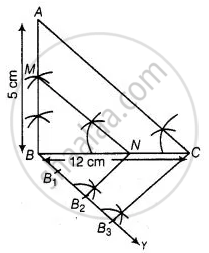# Draw a right triangle ABC in which BC = 12 cm, AB = 5 cm and ∠B = 90°. Construct a triangle similar to it and of scale factor 23. Is the new triangle also a right triangle? - Mathematics

Diagram
Sum

Draw a right triangle ABC in which BC = 12 cm, AB = 5 cm and ∠B = 90°. Construct a triangle similar to it and of scale factor 2/3. Is the new triangle also a right triangle?

#### Solution

Steps of construction:

1. Draw a line segment BC = 12 cm,

2. From 6 draw a line AB = 5 cm which makes right angle at B.3. Join AC, ΔABC is the given right triangle.

4. From B draw an acute ∠CBY downwards.

5. On ray BY, mark three points B1, B2 and B3, such that BB1 = B1B2 = B2B3.

6. Join B3 C.

7. From point B2 draw B2N || B3C intersect BC at N.

8. From point N draw NM || CA intersect BA at M. ΔMBN is the required triangle. ΔMBN is also a right-angled triangle at B.

Concept: Division of a Line Segment
Is there an error in this question or solution?

#### APPEARS IN

NCERT Mathematics Exemplar Class 10
Chapter 10 Construction
Exercise 10.3 | Q 2 | Page 116
Share